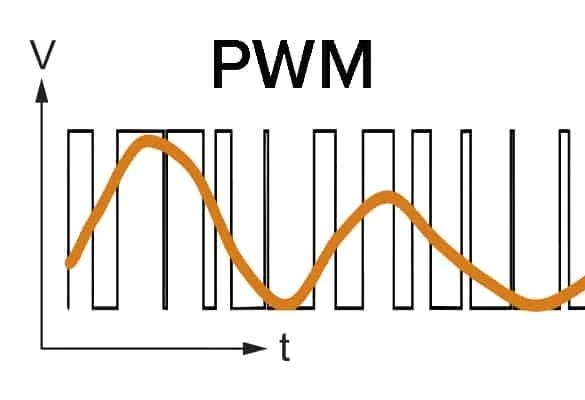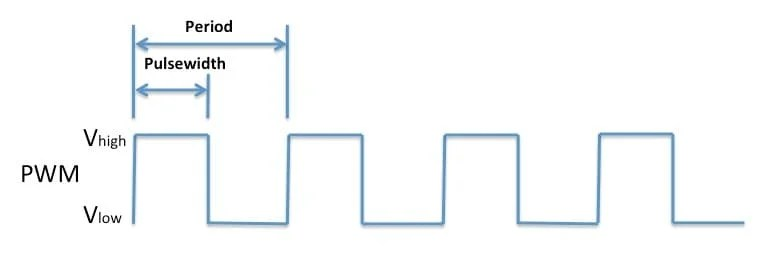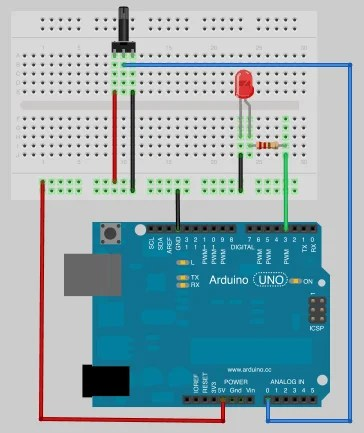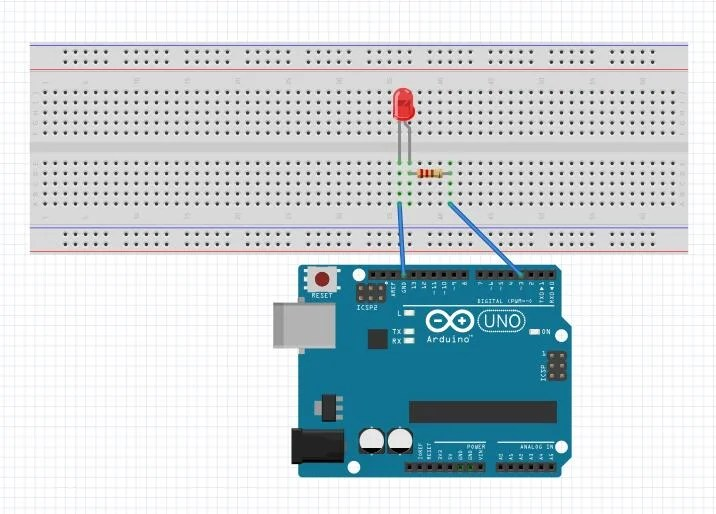View Course Path

# Arduino PWM output and its uses – The definitive guide

In this tutorial, we are going to learn how to perform Arduino PWM (Pulse Width Modulation). We will take a look at how PWM is generated in an Arduino and also try out a couple of applications like dimming an LED and running some motors. Let’s begin!

Contents

## What is Arduino PWM?Pulse width modulation or pulse duration modulation is a technique where we vary the width of a square pulse to control the power supplied to any connected device. Using this technique, we can simulate an analog output using a digital output.

We are using digital control to produce a square wave. This square wave is switched between On (digital high) and Off (digital low). Those are the only two options available to us since it is a digital signal. So how can we attain the full range of discrete analog values using something digital that can have only two values (High and Low)? Well, you forget an essential parameter. Time; we can use Time to introduce the variation.

Using the time for which a pulse is HIGH and LOW, we will achieve our desired result of creating a fake analog signal. To achieve this, we need to acquaint ourselves with two parameters of a PWM signal: Duty cycle and frequency.

## What is the Duty Cycle of a PWM wave?A duty cycle is also known as a power cycle that is the fraction of one period in an active signal. That’s a bunch of complex words. Here’s what a duty cycle means in simple terms. The duty cycle is the percentage of the ratio of the pulse width of the signal to the total period (T). Here’s a formula to further simplify things:

% Duty cycle = (TON/(TON + TOFF))

For example, if a pulse with a period of 20ms remains ON (high) for 4ms, its duty cycle will be

Duty cycle(D) = 4ms / 20ms = 20%

The PWM helps us to control the power that is delivered to the load by using zeroes and ones as on and off signal. Therefore, we can use it to control the motor rotation speed and also the intensity of the LED.

## What is Frequency?Frequency is defined as the number of oscillations or occurrences of a repeating wave per unit time. The period is the duration of the time of one complete cycle. Therefore the period is the reciprocal of the frequency. We can calculate the frequency using the following formula.

frequency = 1 /Time Period.

With regards to PWM, the frequency of the pulses relates to how quickly/slowly, we can get the pulse to the output device. Let’s take an example.

If I am using PWM to light up LED lights, and I want slow and gentle dimming lights- kind of like the ones that light up real slow that people use for romantic dates. Then the frequency of the train of pulses sent to light up the LED will be low.

However, suppose I wish to keep the light continuously ON or flash faster like an ambulance light. Then I can simply increase the frequency of the output, and it will switch ON and OFF so quickly that it will appear to be continuously on to the human eye.

Now you may ask, if I wanted a continuously ON LED, why can’t I use a normal output? And if that’s the question that comes up in your mind, then I salute your concentration. The answer is, with PWM, I can also control how bright I want the lights to be. With digital output, I am stuck between two levels of brightness. 0 or 1. With the analog option (or simulated analog to be pedantic), I have more options in terms of brightness control.

So to summarize:

Duty cycle: Used to control how much power is sent across the device.

Frequency: Used to control the rate at which this power is sent.

## How to calculate Arduino PWM?We can determine the PWM output voltage by using the following formula,

PWM voltage=(Duty cycle ÷ 256) x 5 V.

256 because akin to the 0 to 1 levels available with digital signals, we have 256 levels of analog values that we can work with. 5V because that’s the maximum power you can send via an Arduino Uno.

## What are the uses of pulse width modulation?

• Voltage regulation uses Pulse width modulation.
• A smart lighting system uses PWM to control the brightness.
• We can use Arduino PWM to control the speed of the DC motor.
• Likewise, you can control the degree of rotation of a servo motor using PWM signals.
• Computers use PWM signals in their motherboard that controls the heat generated.
• In addition to that, we can also use it to control various devices such as pneumatic devices like pumps, valves, hydraulics, etc.
• You can use it for dimming an LED.
• You can use it to generate audio signals.

## Why is Arduino PWM used?

A pulse width modulation signal is a type of analog modulation signal. We use it to generate analog signals (make-believe) using the digital signals as input. Arduino PWM signals have a wide range of control applications.

## What are the PWM output pins on Arduino?There are six PWM pins in Arduino Uno. Arduino Uno has 8-bit PWM channels. These PWM pins are represented by the symbol ‘~’. That symbol tells us that these pins have PWM support. The Arduino PWM pins are 3,5,6,9,10 and 11. On these PWM pins, the duty cycle of the PWM pulse, which is nearly 500 Hz, is controlled by the analogWrite function. therefore, the period required is 2ms for the frequency 500hz.

#### Frequency

PWM 3, 5, 6, 9, 10, 11 All pins except 5 and 6 have 490 Hz frequency. Only pins 5 and 6 have 980 Hz frequency.

## Is the Arduino PWM output analog?

The Arduino doesn’t have a built-in digital-to-analog converter (DAC). The ‘analog signals’ are, in reality, pulse width modulated digital signals. You can use the inbuilt function, analogWrite(pin, value), to give a PWM output signal. It has two arguments; they are the PWM output pin, and the other one is the value that is proportional to the duty cycle of the signal.

The signal is off when the value is 0, and the signal is on when the value is 255.

## What are the Arduino functions that deal with PWM signals?

These function does not require any special header file. It reads the value from the declared PIN. Arduino UNO contains a 10-bit analog to digital converter. Therefore, it can represent the value that will map input voltages between 0 and the operating voltage that may be 5v or 3.3v and converts it to the integer values that is ranging between 0 and 1023. Arduino Uno has a resolution between 5v/1024 units that is 4.9mV per unit.

#### Development board

Operating voltage

#### Maximum resolution

Arduino Uno 5 Volts A0 to A5 10 bits

#### Parameters

Integer data type.

pin: The name of the analog input pin that feeds the input (A0 to A5).

#### Returns

Returns the analog value that is fed by the pin.

Data type: integer.

### AnalogWrite():

AnalogWrite() function is also a built-in function that doesn’t require any special header file.

Gives an analog value to the pin that is PWM wave. When we call the analogWrite() function, the pin will generate a steady rectangular wave of the specified duty cycle that remains constant until the next call to analogWrite() on the same pin.

#### Syntax

analogWrite(int pin,int value)

#### Parameters

Pin: The Arduino write pin.

data types: int.

value: It is the duty cycle ranging between 0 (always off) and 255 (always on).

Return no value

### Controlling the Brightness of an LED using a potentiometer

We can control the brightness of the LED using a potentiometer. you can use the analogRead() function to read a voltage and the analogWrite() function to give the output a PWM signal.

#### Hardware Required

• Potentiometer
• LED
• Resistor
• Arduino Uno R3
• Jumper wires

#### Connections:You can rotate the potentiometer, which will change the voltage on pin A0. Using the program, you can change the duty cycle of the PWM signal on digital pin 2, which will adjust the brightness of the LED.

Without a potentiometer, you can connect an LED with pin 2 of the Arduino. For changing the LED’s brightness, our program changes the duty cycle of the PWM signal output at pin 2 of the Arduino.## Code for controlling the brightness of an LED and Arduino’s PWM output (using a potentiometer)

```const int pwm = 3 ;

const int a = 0 ;

void setup()

{

pinMode(pwm,OUTPUT) ;        //setting pin 2 as output

}

void loop()

{

a = map(a, 0, 1023, 0, 255);

/*

the above funtion scales the output of a, which is 10 bit and gives values btw 0 to 1023, in values btw 0 to 255 form analogWrite funtion which only receives  values btw this range

*/

analogWrite(pwm,a) ;

}```

## Code for controlling the brightness of an LED and Arduino’s PWM output

```const int pwm = 2 ;

void setup()

{

pinMode(pwm,OUTPUT) ;        //Set pin 2 as output

}

void loop()

{

analogWrite(pwm,25) ;                 //setting pwm to 25

delay(50) ;                           //delay of 50 ms

analogWrite(pwm,50) ;

delay(50) ;

analogWrite(pwm,75) ;

delay(50) ;

analogWrite(pwm,100) ;

delay(50) ;

analogWrite(pwm,125) ;

delay(50) ;

analogWrite(pwm,150) ;

delay(50) ;

analogWrite(pwm,175) ;

delay(50) ;

analogWrite(pwm,200) ;

delay(50) ;

analogWrite(pwm,225) ;

delay(50) ;

analogWrite(pwm,250) ;

}```

## Controlling the speed of a DC motor using Arduino’s PWM output

We have a detailed article explaining the speed control of DC motor using PWM signals. Please read this article to know how to interface a DC motor with the Arduino board using the motor driver.

## Controlling the speed of a servo motor using Arduino’s PWM output

We have another detailed post explaining the interfacing and control of a servo motor using PWM signals from an Arduino Uno.

This site uses Akismet to reduce spam. Learn how your comment data is processed.

Top